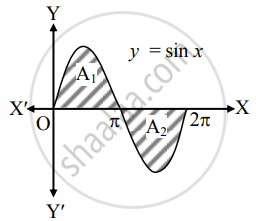# Find the area enclosed between the X-axis and the curve y = sin x for values of x between 0 to 2π - Mathematics and Statistics

Sum

Find the area enclosed between the X-axis and the curve y = sin x for values of x between 0 to 2π

#### Solution

Let A be the required area.

Consider the equation y = sin x.A1 = int_0^"a" sin x  "d"x

= [- cos x]_0^pi

= – (cos π – cos 0)

= – (–1 – 1)

= 2

A2 = int_pi^(2pi)sinx  "d"x

= [- cos x]_pi^(2pi)

= –[1 – (– 1)]

= – 2

∴ A = A1 + |A2|

= 2 + |(– 2)|

= 4 sq.units

Concept: Area Between Two Curves
Is there an error in this question or solution?